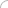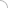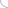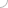# Chemistry interview questions and answers

## Chemistry interview questions and answers

### Chemical thermodynamics interview questions and answers 1) State the third law of thermodynamics. Give its limitations and importance. 2) Explain the laws of Thermodynamics? 3) Explain the following terms: Isolated system, Open system and closed system and give example where ever possible? 4) Give the relation between heat of reaction at constant pressure and that at constant volume? 5) Write a short note on Gibbs Free Energy and derive the equation for the same? Atomic structure interview questions and answers 1) State Heinsenberg’s uncertainty principle. 2) Explain quantum numbers and the different types of quantum numbers present? 3) State different rules used for filling of orbitals in atoms? Chemical kinetics interview questions and answers 1) Explain rate of reaction and the various factors affecting the reaction rate? D block elements interview questions and answers 1) What are d-block elements? Give the properties of d-block elements? 2) Why are complexes of the d-block elements coloured? 3) What are transition metals? Why are Scandium and Zinc not counted as transition metals even though they belong to the d-block? Electrochemistry interview questions and answers 1) Write a short note on standard or reference electrode. 2) Explain the classification of electrodes? 3) What are the advantages and disadvantages of a glass electrode? 4) Write a short note on electrolytic conduction and the various factors affecting it? 5) Write a short note on electrochemical series and give its applications? Ionic equilibria interview questions and answers 1) Explain the definition of Acid and Bases according to Bronsted- Lowry Theory, Arrhenius Theory and Lewis Theory? 2) What do you mean by a Buffer solution? Explain Acidic and Basic buffer with an example? Nuclear chemistry interview questions and answers 1) Classify the nuclides based on the number of protons and neutrons of the nuclei? 2) Give the general characteristics of radioactive decay. 3) Explain the use of radioisotopes in agriculture? Solutions and colligative properties interview questions and answers 1) Explain the following terms: Molality, Mole fraction, Normality, Molarity, Strength, Mass fraction. 2) Write a short note on ideal and non-ideal solutions. 3) What are the different osmotic processes taking place in plants and animals? 400+ Chemistry questions Nuclear chemistry test questions Atomic structure and chemical bonding test questions Solid state chemistry test questions Chemical kinetics test questions Chemical thermodynamics and energetics test questions Solutions and colligative properties test questions D block elements test questions Electrochemistry test questions Ionic equilibria test questions 1. Differentiate between modern periodic table and Mendeleevs table. 2. Explain substituted hydrocarbon. 3. Explain extraction of ephedrine from a mineral block. 4. Write the equation of photosynthesis. 5. Explain the process of spontaneous combustion. 6. Which wavelengths are visible to human eye? 7. How many electrons does benzene have? 8. What is glacial acetic acid? What are its uses? 9. Do all explosions produce carbon dioxide or carbon monoxide? 10. How is arsenic produced? 11. Explain a.) Acid gas b.) Sour Gas 12. Why is acetic acid less conductive than Hcl? 13. Write the chemical formula for Epsom salts? 14. What is protein - according to chemistry? 15. What is the chemical formula of alum? 16. What is polar HCL? 17. Explain the structure of a DNA molecule. 18. Differentiate between organic and inorganic chemistry (adsbygoogle = window.adsbygoogle || []).push({}); Write your comment - Share Knowledge and Experience (adsbygoogle = window.adsbygoogle || []).push({}); (adsbygoogle = window.adsbygoogle || []).push({});Chemistry QuestionsLatest MCQs » General awareness - Banking » ASP.NET » PL/SQL » Mechanical Engineering » IAS Prelims GS » Java » Programming Language » Electrical Engineering » English » C++ » Software Engineering » Electronic Engineering » Quantitative Aptitude » Oracle » English » Finance Home | About us | Sitemap | Contact us | We are hiring © Copyright 2016. All Rights Reserved. Terms of use  |  Follow us on Facebook!